Truncated octahedron

﻿
Truncated octahedron
Truncated octahedron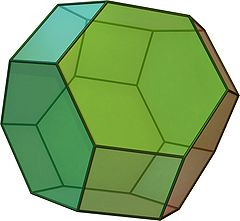Type Archimedean solid
Uniform polyhedron
Elements F = 14, E = 36, V = 24 (χ = 2)
Faces by sides 6{4}+8{6}
Schläfli symbol t0,1{3,4}
t0,1,2{3,3}
Wythoff symbol 2 4 | 3
3 3 2 |
Coxeter-DynkinSymmetry Oh, [4,3], (*432)
Th, [3,3] and (*332)
Dihedral Angle
References U08, C20, W7
Properties Semiregular convex zonohedron
permutohedron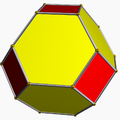Colored faces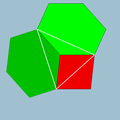4.6.6
(Vertex figure)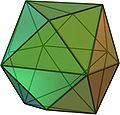Tetrakis hexahedron
(dual polyhedron)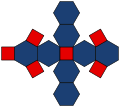Net

In geometry, the truncated octahedron is an Archimedean solid. It has 14 faces (8 regular hexagonal and 6 square), 36 edges, and 24 vertices. Since each of its faces has point symmetry the truncated octahedron is a zonohedron.

If the original truncated octahedron has unit edge length, its dual tetrakis cube has edge lengths$\frac{9}{8}\sqrt{2}$ and$\frac{3}{2}\sqrt{2}$.

Construction

A truncated octahedron is constructed from a regular octahedron with side length 3a by the removal of six right square pyramids, one from each point. These pyramids have both base side length (a) and lateral side length (e) of a, to form equilateral triangles. The base area is then a². Note that this shape is exactly similar to half an octahedron or Johnson solid J1.

From the properties of square pyramids, we can now find the slant height, s, and the height, h of the pyramid:$h = \sqrt{e^2-\frac{1}{2}a^2}=\frac{\sqrt{2}}{2}a$$s = \sqrt{h^2 + \frac{1}{4}a^2} = \sqrt{\frac{1}{2}a^2 + \frac{1}{4}a^2}=\frac{\sqrt{3}}{2}a$

The volume, V, of the pyramid is given by:$V = \frac{1}{3}a^2h = \frac{\sqrt{2}}{6}a^3$

Because six pyramids are removed by truncation, there is a total lost volume of √2 a³.

Coordinates and permutohedron

All permutations of (0, ±1, ±2) are Cartesian coordinates of the vertices of a truncated octahedron centered at the origin. The vertices are thus also the corners of 12 rectangles whose long edges are parallel to the coordinate axes.

The truncated octahedron can also be represented by even more symmetric coordinates in four dimensions: all permutations of (1,2,3,4) form the vertices of a truncated octahedron in the three-dimensional subspace x + y + z + w = 10. Therefore, the truncated octahedron is the permutohedron of order 4.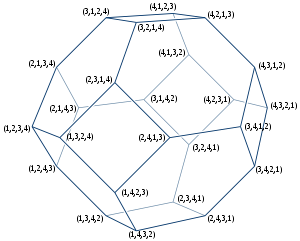Area and volume

The area A and the volume V of a truncated octahedron of edge length a are:$A = (6+12\sqrt{3}) a^2 \approx 26.7846097a^2$$V = 8\sqrt{2} a^3 \approx 11.3137085a^3.$

Uniform colorings

There are two uniform colorings, with tetrahedral symmetry and octahedral symmetry:

Octahedral symmetry Tetrahedral symmetry
(Omnitruncated tetrahedron)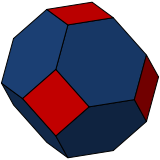122 coloring
Wythoff: 2 4 | 3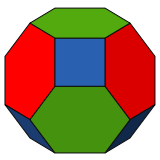123 coloring
Wythoff: 3 3 2 |

Related polyhedra

The truncated octahedron exists within the set of truncated forms between a cube and octahedron:

It also exists as the omnitruncate of the tetrahedron family:

Tessellations

The truncated octahedron exists in three different convex uniform honeycombs (space-filling tessellations):

The cell-transitive bitruncated cubic honeycomb can also be seen as the Voronoi tessellation of the body-centred cubic lattice. The truncated octahedron is one of five three-dimensional primary parallelohedron.

Wikimedia Foundation. 2010.

Look at other dictionaries:

• Truncated cuboctahedron — (Click here for rotating model) Type Archimedean solid Uniform polyhedron Elements F = 26, E = 72, V = 48 (χ = 2) Faces by sides …   Wikipedia

• Truncated icosidodecahedron — (Click here for rotating model) Type Archimedean solid Uniform polyhedron Elements F = 62, E = 180, V = 120 (χ = 2) Faces by sides …   Wikipedia

• Truncated square tiling — Type Semiregular tiling Vertex configuration 4.8.8 Schläfli symbol t0,1{4,4} t …   Wikipedia

• Truncated octahedral prism — Schlegel diagram Type Prismatic uniform polychoron Uniform index 54 Schläfli symbol t0,1{3,4}×{} t0,1,2{3,3}×{} …   Wikipedia

• Octahedron — For the album by The Mars Volta, see Octahedron (album). Regular Octahedron (Click here for rotating model) Type Platonic solid Elements F = 8, E = 12 V = 6 (χ = 2) Faces by sides …   Wikipedia

• Truncated 16-cell — In geometry, the truncated 16 cell or cantic tesseract is a uniform polychoron (4 dimensional uniform polytope) which is bounded by 24 cells: 8 regular octahedra, and 16 truncated tetrahedra. It is related to, but not to be confused with, the 24… …   Wikipedia

• Truncated 24-cell — 24 cell …   Wikipedia

• Truncated rhombic dodecahedron — The truncated rhombic dodecahedron is a convex polyhedron constructed from the rhombic dodecahedron by truncating the 6 (order 4) vertices. The 6 vertices are truncated such that all edges are equal length. The original 12 rhombic faces become… …   Wikipedia

• Truncated 5-cell — 5 cell …   Wikipedia

• Truncated tetrahedron — The truncated tetrahedron is an Archimedean solid. It has 4 regular hexagonal faces, 4 regular triangular faces, 12 vertices and 18 edges. Area and volume The area A and the volume V of a truncated tetrahedron of edge length a are::A =… …   Wikipedia